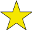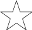## Pillow-Problems: #19

Difficulty:(explanation of difficulty)

The book Pillow-Problems: Thought Out During Wakeful Hours, by Charles Dodgson, better known by the pseudonym Lewis Carroll, was first published in 1893. It contains 72 problems that Carroll thought of while lying awake at night over the course of a few decades. Carroll's intent was that the puzzler would, like he did, work out the answers to the questions mentally. This is problem #19 in the book.
Previous problem: .
Next problem: .

### 19.

There are 3 bags; one containing a white counter and a black one, another two white and a black, and the third 3 white and a black. It is not known in what order the bags are placed. A white counter is drawn from one of them, and a black from another. What is the chance of drawing a white counter from the remaining bag?

[10/87

Answer (in the form of a fraction):

Call the bags A, B, C; so that A contains a white counter and a black one; &c.

The chances of the orders ABC, ACB, BAC, BCA, CAB, CBA, are, a priori,

 (1)/ (6)
each. Since they are equal, we may, instead of multiplying each by the probability it gives to the observed event, simply assume those probabilities as being proportional to the chances after the observed event.

These probabilities are:—

for ABC, ½ ×
 (1)/ (3)
; i.e.
 (1)/ (6)
.
ACB, ½ × ¼; i.e.
 (1)/ (8)
BAC,
 (2)/ (3)
× ½; i.e.
 (1)/ (3)
BCA,
 (2)/ (3)
× ¼; i.e.
 (1)/ (6)
CAB, ¾ × ½ i.e.
 (3)/ (8)
CBA, ¼ ×
 (1)/ (3)
; i.e. ¼

Hence the chances are proportional to 4, 3, 8, 4, 9, 6; i.e. they are these Nos. divided by 34.

Hence the chance, of drawing a white counter from the remaining bag; is

 (1)/ (34)
· {4 × ¾ + 3 ×
 (2)/ (3)
+ 8 × ¾ + 4 × ½ + 9 ×
 (2)/ (3)
+ 6 × ½};
i.e.
 (1)/ (34)
× {3 + 2 + 6 + 2 + 6 + 3}; i.e.
 (22)/ (34)
; i.e.
 (11)/ (17)
.